##### Microeconomics For Dummies - UKIn order to determine the point that maximizes profit, you can determine marginal revenue and marginal cost with calculus. Marginal revenue is the change in total revenue; thus it’s represented as the derivative of total revenue taken with respect to the quantity of output or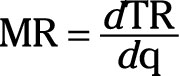Similarly, marginal cost is the change in total cost, so it’s represented as the derivative of total cost taken with respect to the quantity of output produced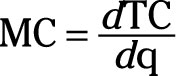The profit-maximizing quantity of output is determined where marginal revenue equals marginal cost.

The market-determined price for your good is \$80. Therefore, your total revenue equals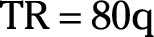Marginal revenue equals the derivative of total revenue taken with respect to quantity or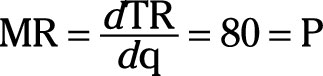Again note that marginal revenue and price are the same in perfect competition.

If your total cost function is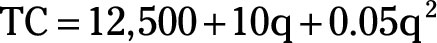Marginal cost equals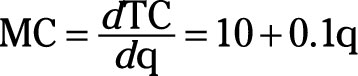In order to determine the profit-maximizing quantity of output, you simply set marginal revenue or price equal to marginal cost and solve for q.

1. Set marginal revenue equal to marginal cost.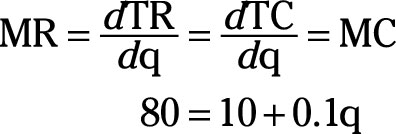2. Solve for q.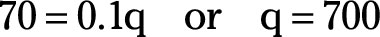The profit maximizing quantity of output is 700 units. Note this is the same answer you obtain when you maximize the total profit equation by using calculus.

Two methods enable you to maximize total profit. First, you can maximize total profit by using total revenue and total cost. Alternatively, you can use marginal revenue and marginal cost to maximize profit.